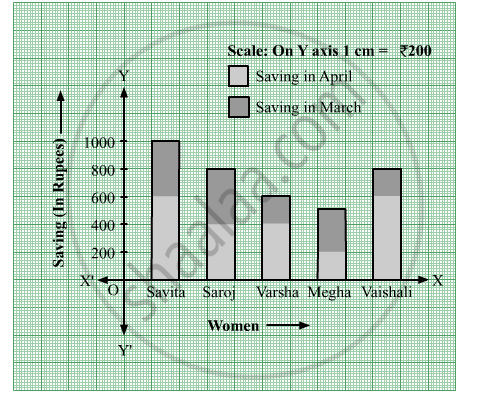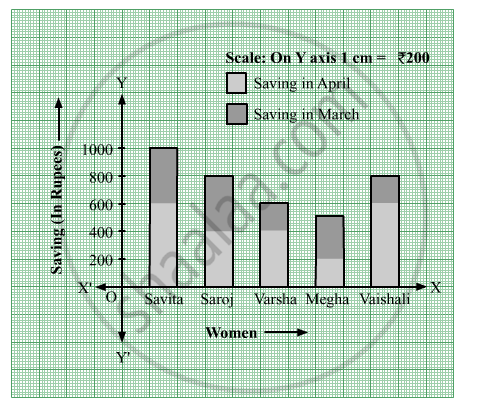# Observe the Following Graph and Answer the Question - Mathematics

Sum

Observe the following graph and answer the question.(1) State the type of the graph.
(2) How much is the savings of Vaishali in the month of April?
(3) How much is the total savings of Saroj in the months March and April?
(4) How much more is the total savings of Savita than the total savings of Megha?
(5) Whose savings in the month of April is the least?

#### Solution(1) This is a bar graph.

(2) The savings of Vaishali in the month of April is Rs 600.

(3) The total savings of Saroj in the months March and April is Rs 800.

(4) Since, the total savings of Savita = Rs 1000, and
The total savings of Megha = Rs 500
Now, 1000 - 500 = 500
So, the total savings of Savita is Rs 500 more than the total savings of Megha.

(5) The savings of Megha in the month of April is the least i.e. Rs 200.

Concept: Subdivided Bar Graph/Diagram
Is there an error in this question or solution?

#### APPEARS IN

Balbharati Mathematics 8th Standard Maharashtra State Board
Chapter 11 Statistics
Practice Set 11.2 | Q 1 | Page 71